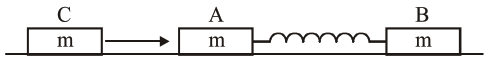# Two identical blocks`
Question:

Two identical blocks $A$ and $B$ each of mass $m$ resting on the smooth horizontal floor are connected by a light spring of natural length $L$ and spring constant $\mathrm{K}$. A third block $\mathrm{C}$ of mass $\mathrm{m}$ moving with a speed $v$ along the line joining A and B collides with A.The maximum compression in the spring is1. $v \sqrt{\frac{M}{2 K}}$

2. $\sqrt{\frac{m V}{2 K}}$

3. $\sqrt{\frac{\mathrm{mv}}{\mathrm{K}}}$

4. $\sqrt{\frac{m}{2 K}}$

Correct Option: 1

Solution:

(1) $\mathrm{C}$ comes to rest

$\mathrm{V}_{\mathrm{cm}}$ of $\mathrm{A} \& \mathrm{~B}=\frac{\mathrm{v}}{2}$

$\Rightarrow \frac{1}{2} \mathrm{is} \quad \mathrm{v}_{\mathrm{ret}}^{2}=\frac{1}{2} \mathrm{kx}^{2}$

$x=\sqrt{\frac{\mu \times v^{2}}{k}}=\sqrt{\frac{m}{2 k}} v$